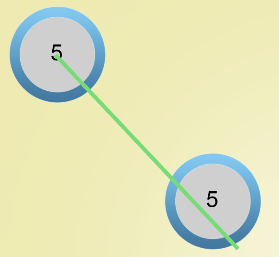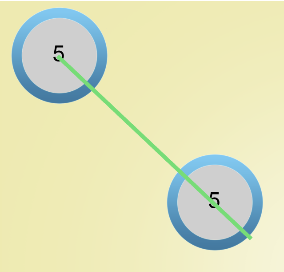# Phaser Calculating A tangent Angles Wrong?

## Recommended Posts

I'm trying to connect two sprites with a line (also a sprite). I tried using the code :

``return this._game.math.angleBetweenY(this._node1.x, this._node1.y, this._node2.x, this._node2.y) * 57.2958;``

This returned a value of 46.68470101066656 and was incorrectly calculating the angle between two objects.

I wrote my own function using JavaScript's Math.atan2 function, like this :

``````calcAngle2() {
let dx = this._node1.x - this._node2.x;
let dy = this._node1.y - this._node2.y;
let theta = Math.atan2(dy, dx);
theta *= 180 / Math.PI;
return theta + 180;
}``````

This returned a value of 43.31531568210369. Please see the attached images for demo.

Am I using phaser's angleBetweenY incorrectly, or is there an issue when using sprites?

Thanks!##### Share on other sites

Hi, thi is Phaser source for angleBetweenY:

``````    /**
* Find the angle of a segment from (x1, y1) -> (x2, y2).
* Note that the difference between this method and Math.angleBetween is that this assumes the y coordinate travels
* down the screen.
*
* @method Phaser.Math#angleBetweenY
* @param {number} x1
* @param {number} y1
* @param {number} x2
* @param {number} y2
* @return {number} The angle, in radians.
*/
angleBetweenY: function (x1, y1, x2, y2) {
return Math.atan2(x2 - x1, y2 - y1);
}``````

You have switched x and y arguments - your method is like angleBetween(), not angleBetweenY().

Regarding results: 46.68470101066656 + 43.31531568210369 = 90